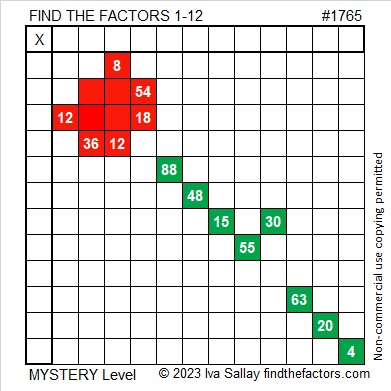# 1765 On This Memorial Day

Contents

### Today’s Puzzle:

This weekend I laid a bouquet of red and white flowers on my husband’s grave and decided to make a red rose Memorial Day puzzle for the blog as well. It is a mystery-level puzzle.

Write the number from 1 to 12 in both the first column and the top row so that those numbers are the factors of the given clues. There is only one solution.### Factors of 1765:

• 1765 is a composite number.
• Prime factorization: 1765 = 5 × 353.
• 1765 has no exponents greater than 1 in its prime factorization, so √1765 cannot be simplified.
• The exponents in the prime factorization are 1 and 1. Adding one to each exponent and multiplying we get (1 + 1)(1 + 1) = 2 × 2 = 4. Therefore 1765 has exactly 4 factors.
• The factors of 1765 are outlined with their factor pair partners in the graphic below.### More About the Number 1765:

1765 is the sum of two squares in two different ways:
42² + 1² = 1765, and
33² + 26² = 1765.

1765 is the hypotenuse of FOUR Pythagorean triples:
84 1763 1765, calculated from 2(42)(1), 42² – 1², 42² + 1²,
413 1716 1765, calculated from 33² – 26², 2(33)(26), 33² + 26²,
1059-1412-1765, which is (3-4-5) times 353, and
1125-1360-1765, which is 5 times (225-272-353).

1765 is a digitally powerful number:
1⁴ + 7³ + 6⁴ + 5³ = 1765.

1765 is a palindrome in a couple of different bases:
It’s A5A base 13 because 10(13²) + 5(13) + 10(1) = 1765, and
it’s 1D1 base 36 because 1(36²) + 13(36) + 1(1) = 1765.

## 2 thoughts on “1765 On This Memorial Day”

1.Charles Sanders

How did you ever decide to explore a number in base 36?

2.ivasallay

I made a table. It goes to base 100, but I could easily make it go well beyond that if I wanted. I don’t always look very far down on the table when I write a post. When I wrote this one, I typed 1765 into the table, and it told me what that number was in several other bases.1765 is also 101 in base 42.

This site uses Akismet to reduce spam. Learn how your comment data is processed.